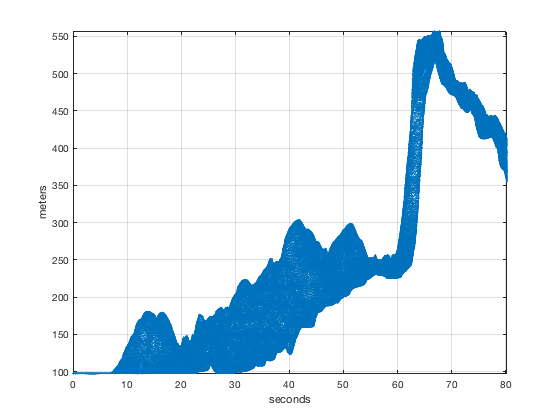# hfive2struct documentation

h5struct loads HDF5 data into a Matlab stucture with the same hierarchy as the original file.

## Syntax

```data = hfive2struct(filename)
data = hfive2struct(filename, dataset)
data = hfive2struct(filename, dataset, true)
data = hfive2struct(filename, [], true)```

## Description

data = hfive2struct(filename) loads all attibutes, dataset values and dataset atributes of the h5 filename into a Matlab stucture with the same hierarchy as the original file. [currenlty handles up to 11 group levels]

data = hfive2struct(filename, dataset) loads only the requested dataset and associated attibutes of filename into a Matlab stucture with the same hierarchy as the original filename. dataset is the full h5 path to the desired dataset. dataset can be specified as either a string or cell array of stings e.g. /path/to/dataset or {path/to/dataset1, path/to/dataset2}.

data = hfive2struct(filename, dataset, fill_with_nan) loads only the requested dataset and associated attibutes of filename into a Matlab stucture. If fill_with_nan = true, all single and double datasets having a _FillValue will have _FillValue replaced with NaNs.

## User Input

• filename full path to hdf5 file
• dataset full dataset path within hdf5 structure (optional). Can be specified as either a string or cell array of stings e.g. '/orbit_info/rgt' or {'/orbit_info/rgt', /ancillary_data/control'}.
• fill_with_nan true or false, if true single and double datasets with a _FillValue will have _FillValue filled with NaN.

## Example 1

Load in all data from an h5 file:

```D = h5struct('altimetry_example.h5');
```

Now here are the structured contents of D

```D
```
```D =
struct with fields:

elevation: [1×1 struct]
latitude: [1×1 struct]
longitude: [1×1 struct]
Attributes: [1×1 struct]
ancillary_data: [1×1 struct]
instrument_parameters: [1×1 struct]
```

To access one of the fields of D, follow D with a . and then the field name. For example, to access the elevation data, do so like this:

```D.elevation
```
```ans =
struct with fields:

Value: [236379×1 single]
Attributes: [1×1 struct]
```

The elevation measurements reside in the D.elevation.Value field, and information about the elevation data can be found in D.elevation.Attributes. Here are the attributes of the elevation data:

```D.elevation.Attributes
```
```ans =
struct with fields:

h5es_id: 4
units: 'meters'
long_name: 'elevation'
standard_name: 'elevation'
description: 'Elevation of the laser spot above ellipsoid'
```

Plot elevation and signal strength as a 3D scatterplot:

```figure
scatter3(D.longitude.Value, D.latitude.Value, D.elevation.Value,...
2, D.instrument_parameters.rcv_sigstr.Value,'filled')

% Add a grid and some labels:
grid on
xlabel(D.longitude.Attributes.units, 'interpreter', 'none');
ylabel(D.latitude.Attributes.units, 'interpreter', 'none');
zlabel(D.elevation.Attributes.units, 'interpreter', 'none');

cb = colorbar;
ylabel(cb,D.instrument_parameters.rcv_sigstr.Attributes.long_name)
axis tight
```## Example 2

If you only need some of the data in an HDF5 file, you may prefer to only load the data Here's how:

```dataset = {'/instrument_parameters/rel_time', '/elevation'};
D = h5struct('altimetry_example.h5', dataset);
```

This time we only loaded the elevation data and the relative time. Here's what D looks like:

```D
```
```D =
struct with fields:

instrument_parameters: [1×1 struct]
elevation: [1×1 struct]
```

Similar to Example 1, plot elevation vs. relative time

```figure
plot(D.instrument_parameters.rel_time.Value, D.elevation.Value,'.','markersize',0.5)

% add a grid and some labels
grid on
xlabel(D.instrument_parameters.rel_time.Attributes.units, 'interpreter', 'none');
ylabel(D.elevation.Attributes.units, 'interpreter', 'none');
axis tight
```## Author Info

This function and supporting documentation were written by Alex S. Gardner, JPL-Caltech, Oct 2018.